# Making a Möbius strip

Sophie Bleau chops up, unravels, and squeezes the torus into shapeSo, how do you make a Möbius strip? You take a strip of paper, twist an edge and join up the edges. Tadaaahhh! End of article.

Just kidding. But this shape is indeed a Möbius strip, and it has some interesting properties. For instance, if you cut the Möbius strip down its centreline and unfold your new creation you will find that you have made a surprisingly untwisted (if slightly large) paper ring. But, as cool as Mr Möbius is, it’s his relationship with Mrs Torus that I really want to talk about. Before I delve in, I will first give a disclaimer that we are about to enter the area of topology, which is inherently a rather flexible field, in that one can stretch, bend, cut and glue topological spaces, and have them still be considered… well, the same! The ability to cut and glue things comes with a caveat: two things that have been cut apart must be glued back together in exactly the same way when we’re done stretching and bending. We’ll come back to what this means later. The ability to stretch things out in topology is what I like to call the stretchiness axiom. For instance, these four shapes are topologically equivalent: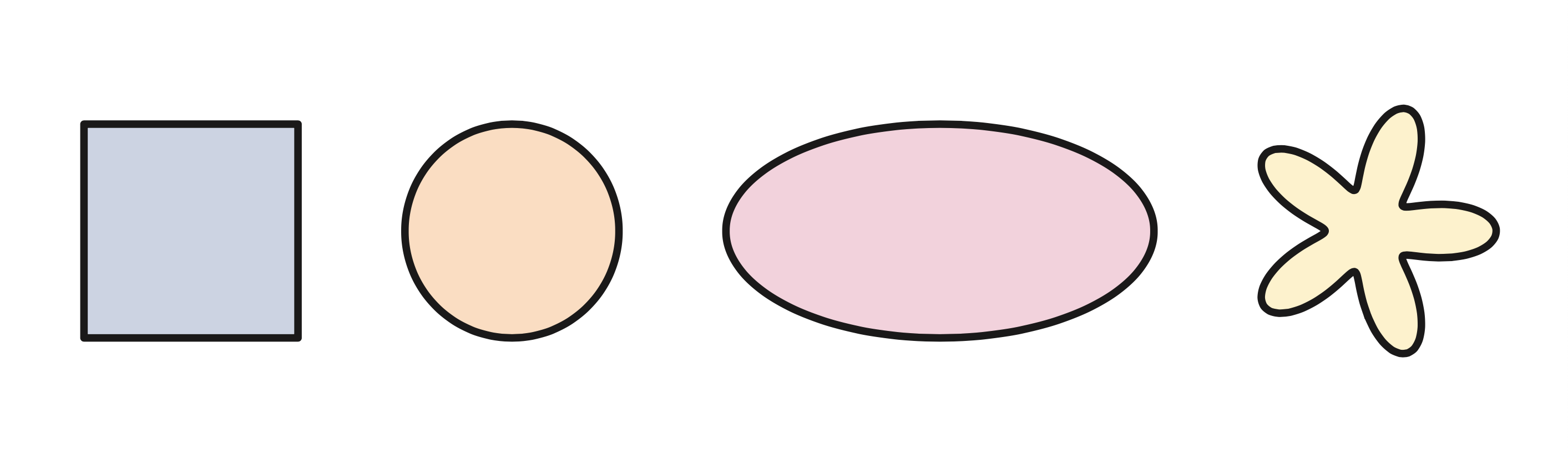whereas this,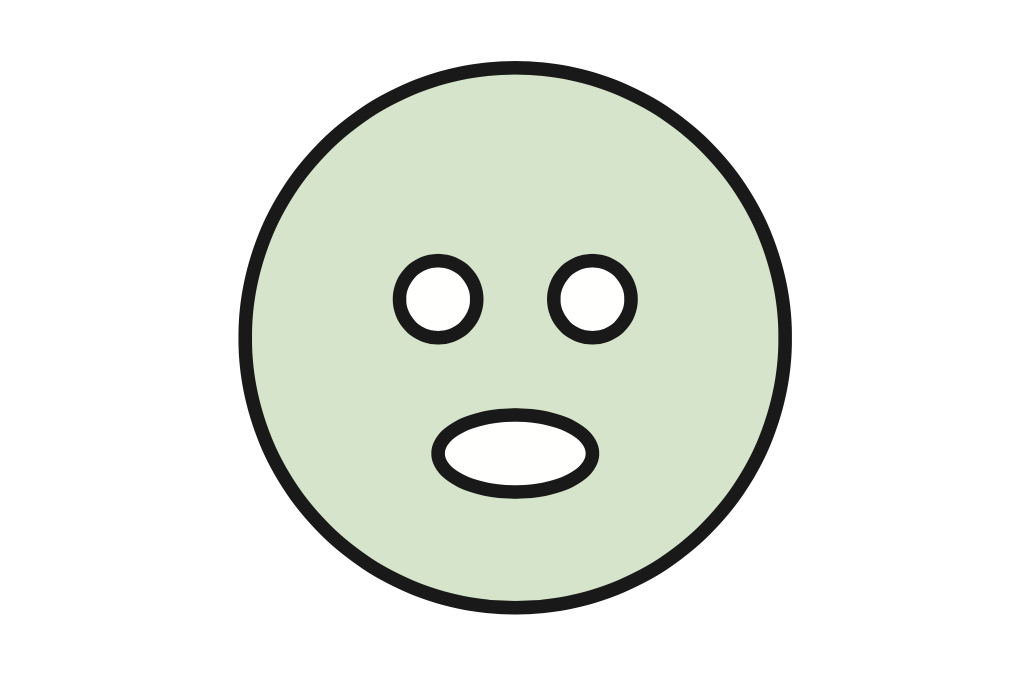is not equivalent, since there is no way to bend the shape to fill in the holes. See? Pretty tangible stuff we’re dealing with. However today our topology is going to get uncharacteristically nitpicky. So without further ado, let us see whether the torus actually is the Möbius strip.

Our torus is the surface of a doughnut in shape. So if we slice through one side of it and stretch it straight, as shown in the diagram below, we’ll get another shape we know quite well: the cylinder. Then, slicing the cylinder down the side, we can fold it out to obtain a rectangle, which is basically a square in topology language (stretchiness axiom activated). This is to say that, if we remember the top edge matches with the bottom edge (to make the cylinder), and the left edge matches with the right edge (to make the torus) then our square is topologically equivalent to the torus. By invoking again the stretchiness axiom, we can rescale this to get the unit square, of side length 1. We use arrows in these diagrams to remind us what matches with what, and with which orientation. This is important, since if we end up cutting the Möbius strip and forgetting which way it used to be attached, we could untwist and reglue it any way we want. Untwisting it would make it into a cylinder, which certainly isn’t topologically equivalent to Mr Möbius.

As we can see in the diagram below, the punchline is a square. Imagine this square to be populated with coordinate points of the form $(a,b)$, where the order of the pair influences where the point is. We call this the space of ordered pairs.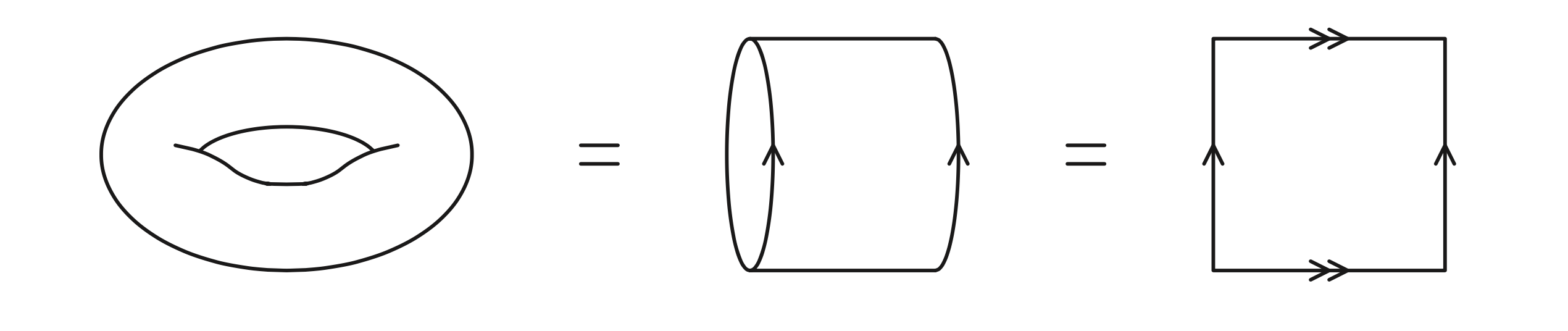To state formally what it means to identify the arrows, we can imagine each line as an interval from $0$ to $1$. Then every point in the square has coordinate $(a,b)$ for $a$ and $b$ between $0$ and $1$. The identification then means that a point $(x,0)$ on the bottom double arrowed line is the same as the point $(x,1)$ on the top double arrowed line, and that a point $(0,x)$ on the left single arrowed line is the same as the point $(1,x)$ on the right single arrowed line. These are the constraints on the square to obtain the torus.

Now, I know what you’re thinking. This isn’t nitpicky at all. In fact, for a mathematician, I seem to be curiously notpicky. So I give it to you here. Let’s take the unit square with the left-right edge identification and the top-bottom edge identification (remembering the orientations as shown in the picture), and add an additional constraint: a point $(a,b)$ in the unit square must be identified with the point $(b,a)$. In other words, we’re restricting the space of ordered pairs to the space of unordered pairs. You may think that this constraint is more flexible, even reckless! Not caring the order of the entries in a tuple is indeed madness. However in order to forget about the order of entries in a tuple—or in this case, a pair—we must make sure that everything matches up. This final constraint may indeed put a spanner in the works with its diva-level high demands, but we put in the effort to catch a glimpse of the glory that is the configuration space. A configuration space on $n$ elements is the space of unordered $n$-tuples, and here we are looking at the configuration space on 2 elements. This kind of space is one which comes up here and there in topology, and is a simple yet fruitful object of study. Furthermore, fixing the configuration constraint here may give us a surprisingly nice result.

In case you’ve lost track of all the constraints, let’s list them:

• for any point on the top or bottom edge, we have $(t,0)\sim(t,1)$,
• for any point on the left or right edge, we have $(0,l)\sim (1,l)$,
• for any point in the square, we have $(a,b)\sim(b,a)$,

where $\sim$ denotes identification. The first two of these constraints define the torus, but what shape forms when we add this extra one? The easiest way to enact the last constraint is to recognise which points match up: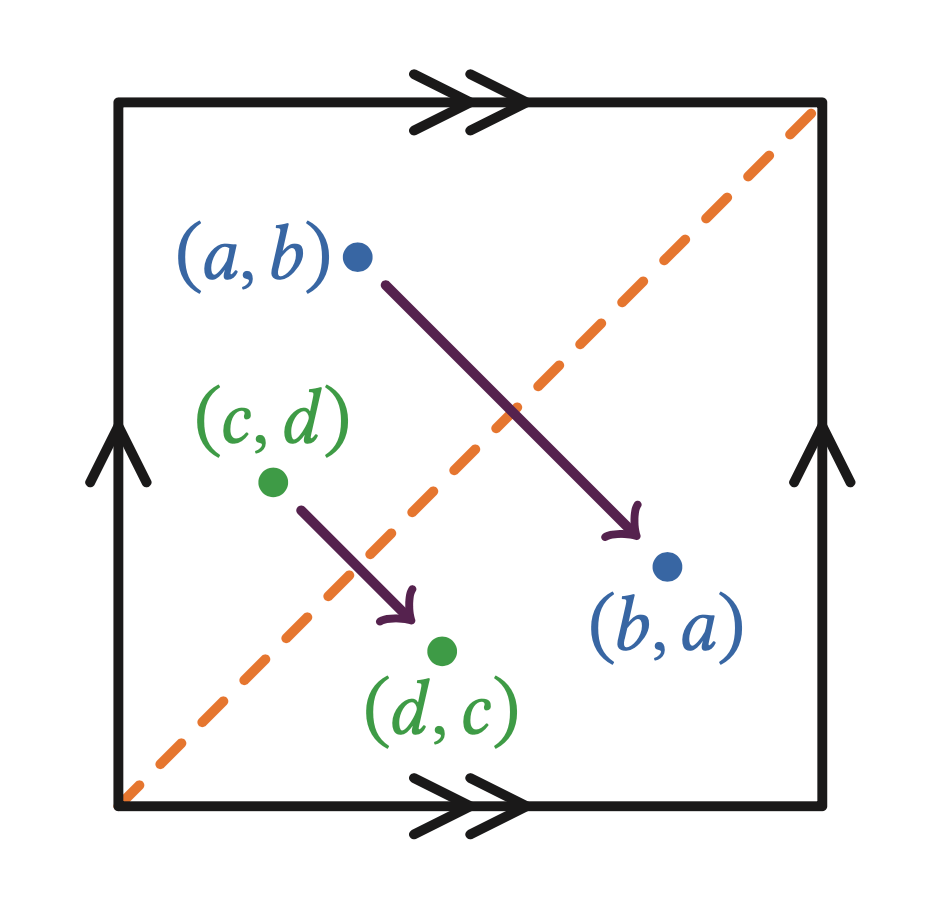We can see that the points in the triangle below the diagonal correspond exactly to those above the diagonal. As the points above the diagonal add no new information, we fold down along the diagonal to make a right-angled triangle. We don’t really need the points on the diagonal at the moment (ie points of the form $(a,a)$) so let’s delete them—although I’ve highlighted where they would have been in red so we can see what became of them should they need to be salvaged. If I ever need them again, I’ll call them $\Delta$ (for $\Delta$iagonal). But now, by identifying the left edge with both the bottom edge and the right edge (and the top edge with both too), we realise this means we need to glue the horizontal edge to the vertical edge.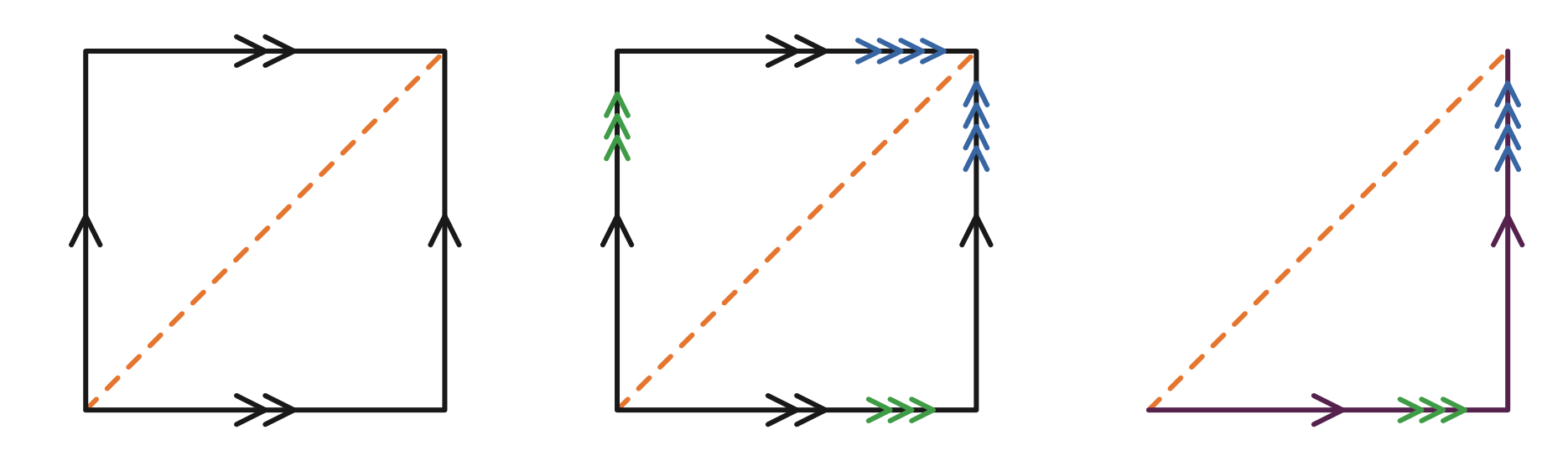The identifications we will make on the torus. The top and bottom edges are identified, the left and right edges are identified, and then all points $(a,b)$ are identified with their diagonal twin $(b,a)$.

This seems a bit impossible. One way of going about it is cutting it. The running theme here is that cutting is allowed as long as you remember to glue the cut back together again in the same place with the same orientation. Indeed, we cut the triangle down the middle and flip one of the pieces to glue the two required edges, as shown above. The pink edges are the ones we have to remember to glue back together in the correct orientation.Gluing the last two edges together seems difficult, but, again due to the fact that we are in the flexible world of topology, we can stretch our grid out to a long strip, and flip while folding so that the two pink arrows are facing the same way. Now we have a Möbius strip; all procured from the space of unordered pairs.Another way to get from the triangle to the Möbius strip is to imagine it’s a mozzarella cheese toastie. Since the cheese is stretchy, you can pull apart the two edges at the right-angle and stretch it out to a rectangle, and then twist and glue the edges from there to make our Mr. Möbius. This is slightly more intuitive for me, but we also lose a bit of information, since the edges (or more accurately, singular edge) of the Möbius strip aren’t as explicitly shown to be the diagonal values $\Delta$.

The method we used in the first place lets us see that including the set $\Delta$ of points $(a,a)$ corresponds to including the edges of the Möbius strip, so we can include them again if we want (although it is customary to leave them out).

The method of identifying points is known as a quotient map, and here we have quotiented the torus by the relation $\sim$ defined by $(a,b)\sim (b,a)$ to get the Möbius strip. Hereon, I’ll refer to the middle square in our torus identifications as the Möbius square.

## The map to the circle

Our study of the Möbius strip is all about turning lines into circles, seeing if we can manipulate things in a fruitful way, turning circles into lines, and doing it all again. With this in mind, we ask ourselves whether it is possible to find a map from the Möbius strip to the circle.

One way to get from the set of all unordered pairs to the circle is simply to use the projection map $\pi_1$ from the pair to its first coordinate; $(x,y) \mapsto x$. However we run into a problem because $(x,y)=(y,x)$ so $(x,y)$ also maps to $y$. This requires that $\pi_1(x,y)=x=y$ which is a contradiction since by removing $\Delta$ we require that $x\neq y$. So we need another idea for our map.

I’m going to ask you to trust me here, because this map might get… weird. First, rotate the Möbius square until the orange diagonal $\Delta$ is horizontal. Also, take the circle and unroll it underneath this diamond, until it’s a straight line of the same length as $\Delta$ (also horizontal). For our map $p$, any point $(a,b)$ in the Möbius square is sent to the point at which the vertical line through $(a,b)$ intersects the line below. This looks promising; for any $(a,b)$ we have $p(a,b)=p(b,a)$, so one of our constraints is satisfied (as $p(a,b)=p(b,a)$ is stronger than the two being equivalent).

Turning our attention to the other constraints, we see that we need the equivalence $p(0,t)\sim p(1,t)$, as in the Möbius square. This is a bit strange, because the the line down from $(0,t)$ is nowhere near the line down from $(1,t)$. Hmm. Okay, let’s try to make progress with the other constraint; here we want $p(t,0)\sim p(1,t)$. And again, we have that the line down from $(t,0)$ is nowhere near the line down from $(t,1)$. That’s not so helpful. Then what can we do with this?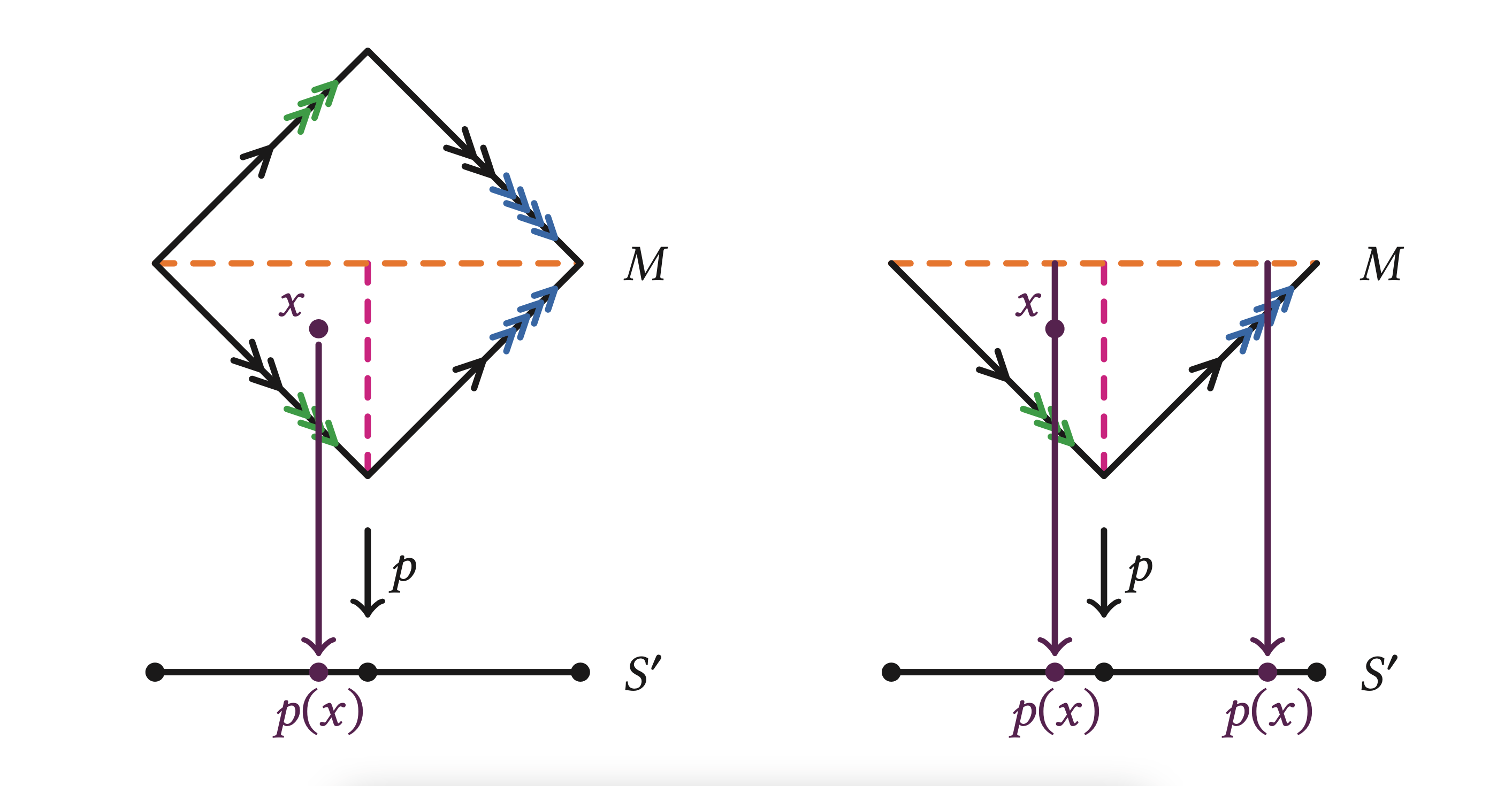Well, I suppose if we pick some $t\in [0,1]$ and draw out the diagram, we can see that the image of $(0,t)$ and $(1,t)$ are exactly a half-line apart. The same is true for $(t, 0)$ and $(t,1)$. So perhaps let us identify not only the start and endpoints of this line representing the circle, but also the midpoint. Then the line is representative of two loops of the circle. Another way to say this is that the map is 2-periodic.

In a way, this makes sense: if we identify $(a,b)$ and $(b,a)$ to be the same then all the information we need is where the line joining them meets our orange diagonal $\Delta$, so really our map $p$ is a projection from $\Delta$ to the circle, where $\Delta$, as we found earlier, represents the edge of the Möbius strip, wrapping twice around the circle. So, since $\Delta$ is a double looped circle, it’s no wonder that the interval we’ve laid out below turns out to be one too. You can imagine that if we squeezed the Möbius strip width-ways until the width was 0 (ie the strip was a single line) then the edge of the Möbius strip would literally run the same circle twice.

With this in mind, let’s reassess. We have a map $p$ from Mr Möbius to the circle with $p(0,t)=p(1,t)$; $p(t,0)=p(t,1)$; and $p(a,b)=p(b,a)$. This loops twice around the circle. Recall that the first two identifications come from the identification of the two single arrows and the two double arrows in the Möbius square. The third identification specifies the unordering of pairs in the Möbius strip, ie reflection in $\Delta$. So the preimage (known in topology as the fibre) of each point in the circle will have two lines in the Möbius square, as shown.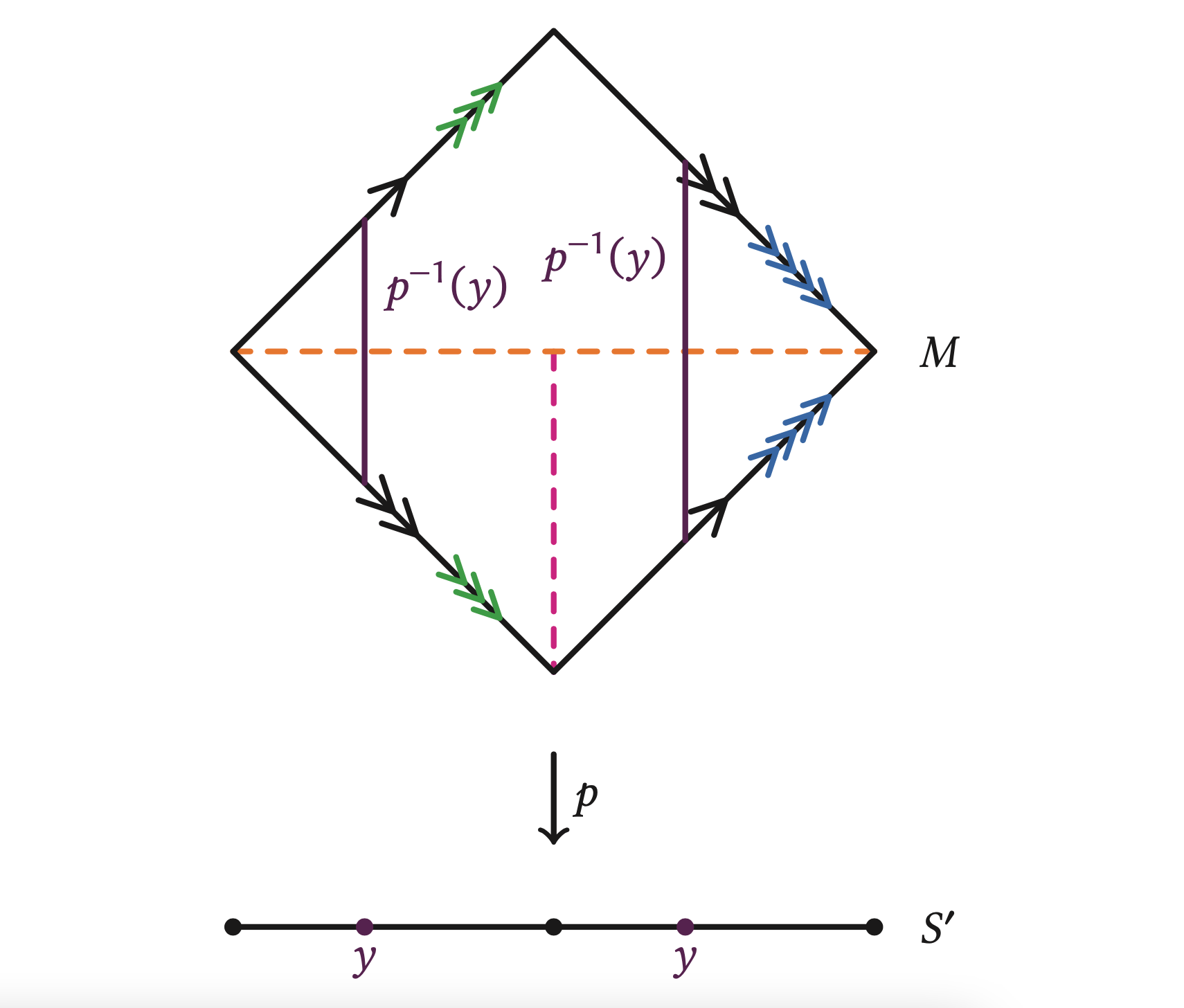This is all well and good, but why can we only seem to find 2-periodic maps; why do we have plenty of maps to a double loop of the circle, and not just the circle itself? To answer this, let’s figure out on the Möbius square where the centreline will be.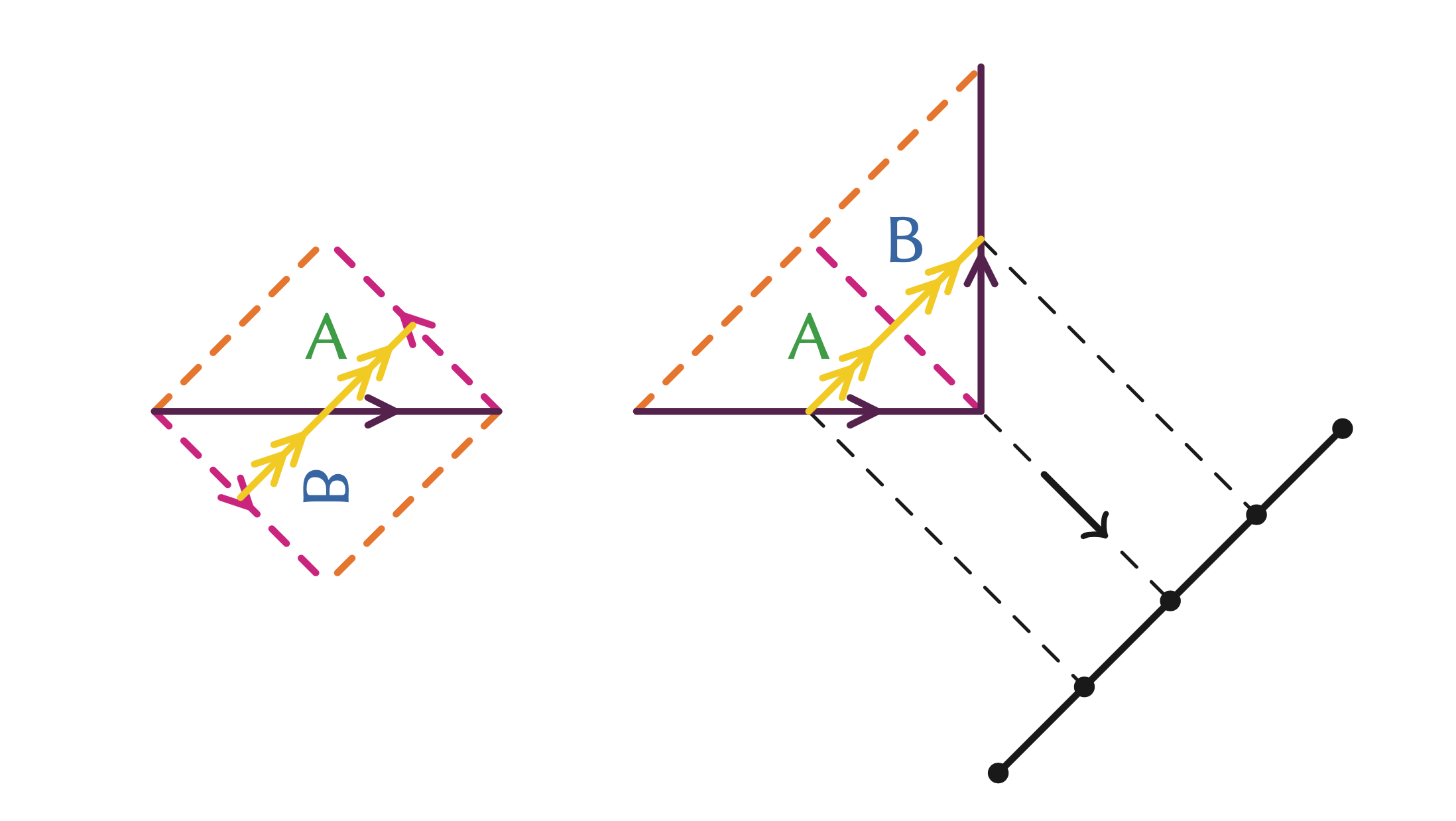This shows that the centreline passes through all the unique points in the image. So, we can imagine that any map which doesn’t pass through the centreline has to map twice around the circle; to stay continuous, we need to stay either in the top half or the bottom half of the Möbius square. To give you an idea of why this restriction would have this effect, we are limited to maps like the one sending the top edge of the Möbius strip to the circle. As we know, following the top edge of the Möbius strip amounts to looping twice around the Möbius strip, since the strip has only one edge. Intuitively, if we imagine instead that we are mapping from the boundary of the Möbius strip to the circle that forms its centreline, we loop twice around it, getting a 2-periodic map. Meanwhile, the map from the centreline (and those paths equivalent to it, except for slight detours) maps to a once-looped circle. We can imagine that a continuous path similar to the centreline except for a slight detour is like a deformation of the centreline. Therefore, any such path will also have a once-looped map to the circle. Having found your long sought-after circle map, you may now put your feet up; our work is done!

In this article, not only have we gained the invaluable skill of making a paper Möbius strip, we have also (con)figured out how to construct it topologically from another shape: the torus. In doing so, we have discovered that the only difference between the two spaces is the specification on ordering, which is an entirely unexpected result. The circle underpinning the theory of the Möbius strip has also been catered for: the map we have found from the Möbius strip down to the once-looped circle was constructed so that we can map back up to the Möbius strip if we want to. Indeed, these maps are those from slight deformations of the Möbius strip’s centreline. This is with the knowledge that straying too far from this lands us on the Möbius square’s diagonal, and the Möbius strip’s edge, which, as we know, loops twice around the circle, and so doesn’t give us a nice two-way map anymore.

I will leave you with a question. Clearly cutting a band and giving it a half twist gives a Möbius strip, which is not topologically equivalent to it. However, an even number of half twists would match up the arrows such that the arrow orientations agree. So, is a band with an even number of half twists topologically equivalent to the untwisted band? Using this, can you conclude whether it is possible to find an $n$-periodic map by taking further half twists of the Möbius strip? I hope that the reader will be enticed to ponder further on this problem, and the many others in the literature surrounding the Möbius strip.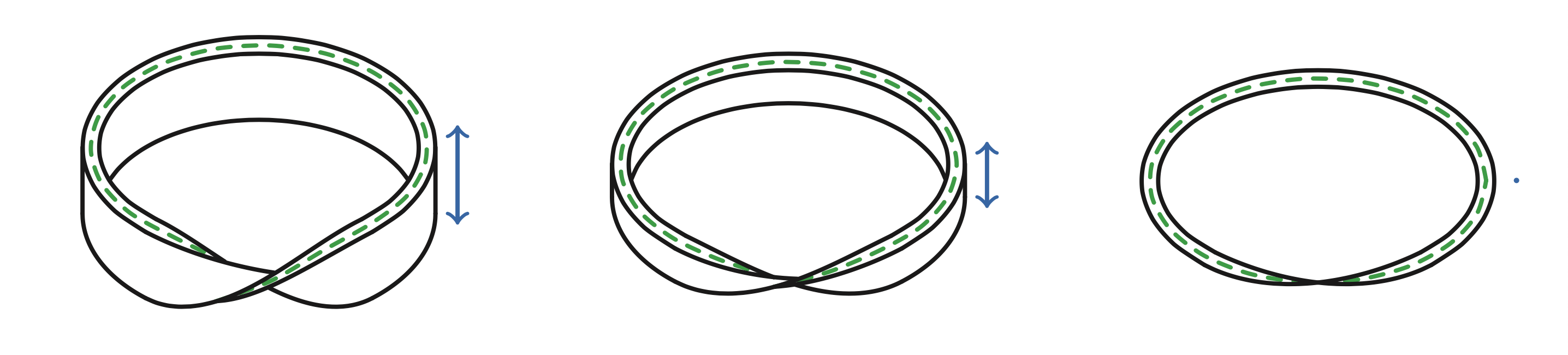Sophie is in the fourth year of her maths degree at the University of Edinburgh. She spends her free time drawing Celtic knots and fangirling over Leonardo da Vinci. She has three cats (if Sophie has n cats, the qualification for being a ‘crazy cat lady’ is always n + 1 cats) called Pushkin, Norma, and Idgie, who take great pleasure disrupting any mathematical progress—usually by sitting on it.